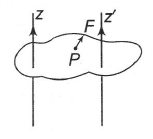Figure shows a lamina in x-.y plane. Two axes z and z’ pass perpendicular to its plane. A force F acts in the plane of lamina at point P as shown. Which of the following statements is incorrect? (The point P is closer to z’-axis than the z-axis).

# Figure shows a lamina in x-.y plane. Two axes z and z' pass perpendicular to its plane. A force F acts in the plane of lamina at point P as shown. Which of the following statements is incorrect? (The point P is closer to z'-axis than the z-axis).1. A

Torque $\mathrm{\tau }$ caused by F about Z axis is along $\stackrel{^}{\mathrm{k}}$

2. B

Torque ${\mathrm{\tau }}^{\text{'}}$ caused by F about ${\mathrm{z}}^{\text{'}}$ axis is along $-\stackrel{^}{\mathrm{k}}$

3. C

Torque caused by F about z axis is greater in magnitude than that about z' axis.

4. D

Total torque is given by

Register to Get Free Mock Test and Study Material

+91

Verify OTP Code (required)

### Solution:

The direction of $\stackrel{\to }{\mathrm{\tau }}$ is given by right handed screw rule.
According to this rule, the direction of $\stackrel{\to }{\mathrm{\tau }}$ caused by $\stackrel{\to }{\mathrm{F}}$ about z axis is along $\stackrel{^}{\mathrm{k}}$.
Hence, option (1) is correct.
Again, according to this rule, the direction of ${\stackrel{\to }{\mathrm{\tau }}}^{1}$ caused by $\stackrel{\to }{\mathrm{F}}$ about z' axis is along -$\stackrel{^}{\mathrm{k}}$
Hence, option (2) is also correct.
As  and P closer z'axis, therefore, ${\stackrel{\to }{\mathrm{\tau }}}^{1}$ caused about z' axis is smaller than $\stackrel{\to }{\mathrm{\tau }}$ .
Hence option (3) is also correct.